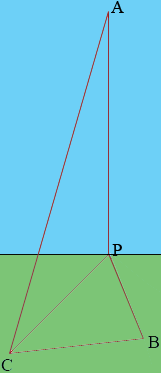SEARCH HOMEMath Central Quandaries & QueriesQuestion from Murtaza: An aircraft is vertically above a point which is 10 km west and 15 km north of a control tower. The aircraft is 4000 m above the ground, how far is it from the control tower?Murtaza,

The subject of your email wa Pythagoras' Theorem. Well you actually get to use Pythagoras' Theorem twice.

In my diagram C is the control tower, A is the airplane, P is the point on the ground directly below the airplane and B is the point on the ground 15 km north of the control tower.Angle CBP is a right angle and you know that the length of CB is 15 km and the length of BP is 10 km so you can use Pythagoras' Theorem to find the length of CP. Now since angle CPA is also a right angle and you know the lengths of CP and PA you can use Pythagoras' Theorem again to find the length of CA. (Watch the units.)

PennyMath Central is supported by the University of Regina and The Pacific Institute for the Mathematical Sciences.